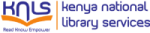Normal view

# Practical scientific and numerical computing with MATLAB and Python / Eihab B.M. Bashier.

Material type:TextPublisher: Boca Raton : CRC Press, 2020Description: 1 online resource (1 volume : illustrations (black and white.)Content type:
• text
Media type:
• computer
Carrier type:
• online resource
ISBN:
• 9780429021985 (electronic bk)
• 0429021984 (electronic bk)
• 9780429664106
• 0429664109
• 9780429666827
• 0429666829
DDC classification:
• 502.85 23
LOC classification:
• Q183.9 .B375 2020
Online resources: Summary: "This book concentrates on the practical aspects of numerical analysis and linear and non-linear programming. It discusses the methods for solving different types of mathematical problems using MATLAB and Python. Although the book focuses on the approximation problem rather than on error analysis of mathematical problems, it provides practical ways to calculate errors. The book is divided into three parts, covering topics in numerical linear algebra, methods of interpolation, numerical differentiation and integration, solutions of differential equations, linear and non-linear programming problems, and optimal control problems"--
Tags from this library: No tags from this library for this title.
Star ratingsAverage rating: 0.0 (0 votes)
No physical items for this record

"This book concentrates on the practical aspects of numerical analysis and linear and non-linear programming. It discusses the methods for solving different types of mathematical problems using MATLAB and Python. Although the book focuses on the approximation problem rather than on error analysis of mathematical problems, it provides practical ways to calculate errors. The book is divided into three parts, covering topics in numerical linear algebra, methods of interpolation, numerical differentiation and integration, solutions of differential equations, linear and non-linear programming problems, and optimal control problems"--